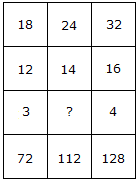# Verbal Reasoning - Character Puzzles - Discussion

### Discussion :: Character Puzzles - Character Puzzles 1 (Q.No.8)

8.

Which one will replace the question mark ?[A]. 2 [B]. 3 [C]. 4 [D]. 5

Explanation:

(18 x 12)/3 = 72

and (32 x 16)/4 = 128

Therefore, (24 x 14)/? = 112

(336/?) = 112

? = (336/112)

? = 3.

 Thangavel said: (Jul 29, 2016) I understand the solution, thank you.

 Sheela said: (Aug 11, 2016) Thank you for giving me a clear idea.

 Niveditha.S said: (May 15, 2018) Thanks for explaining the solution.

 Happy Maan said: (Aug 16, 2018) This question was two solution 1.12÷3=4. 4*18=72. 3.16÷4=4. 4*32=128. 2.14÷2=7. 7*24=168.

 Suvarna said: (Aug 17, 2018) Nice, thanks for explaining it.

 Animesh Dey said: (Jul 6, 2021) Thank you so much for explaining it.

 Bhoomika G R said: (Sep 16, 2021) Very nice. Thank you so much.

 Bhoomika G R said: (Sep 16, 2021) Very nice. Thank you so much.